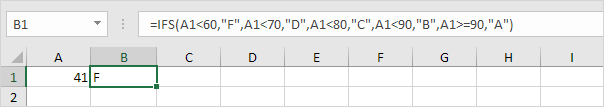# SWITCH

The SWITCH function in Excel looks up a specified value in a list of values and returns the result corresponding to the first match found.

### Simple SWITCH

Let's start simple. The SWITCH function below looks up the value in cell B3.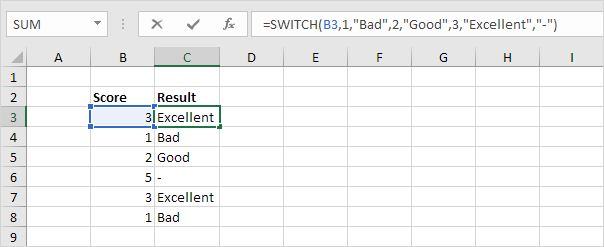Explanation: if the value is 1, the SWITCH function returns "Bad". If the value is 2, the SWITCH function returns "Good". If the value is 3, the SWITCH function returns "Excellent".

The last argument (a dash in this example) is always the default value (if there's no match).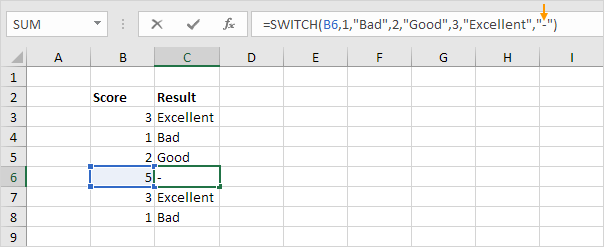Let's kick it up a notch! The SWITCH function below reduces the price of green and blue tables by 50% (see orange arrows). All other products are discounted by 10%.Explanation: the formula shown above uses the & operator to concatenate (join) the string in cell B3 and cell C3. If the value is "TableGreen", the SWITCH function reduces the price by 50%. If the value is "TableBlue", the SWITCH function also reduces the price by 50%. The last argument (10% discount) is always the default value (if there's no match).

### Combine SWITCH with other Functions

Let's combine the SWITCH function with other Excel functions. For example, the SWITCH function below looks up the result of the RIGHT function.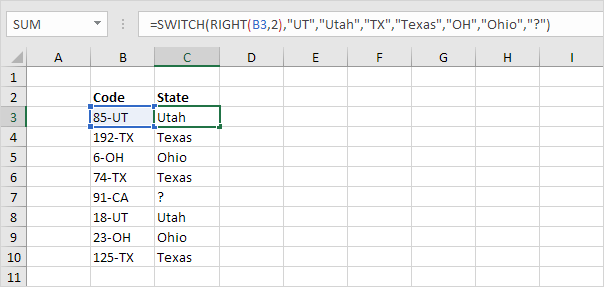Explanation: the RIGHT function extracts the 2 rightmost characters from the string in cell B3. If UT, the SWITCH function returns "Utah". If TX, the SWITCH function returns "Texas". If OH, the SWITCH function returns Ohio. The last argument (a question mark in this example) is always the default value (if there's no match).

### SWITCH or IFS?

The IFS function below produces the exact same result.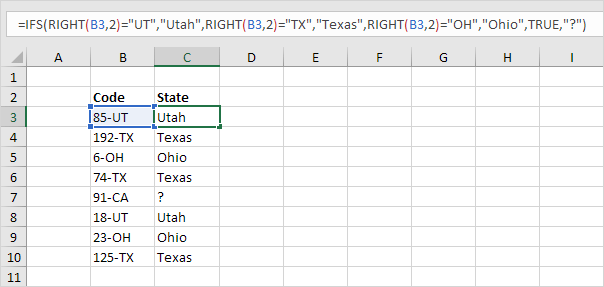Conclusion: the SWITCH function is shorter and easier to read.

However, the SWITCH can only perform an exact match. When using comparison operators like "<" and ">=", use IFS instead of SWITCH.